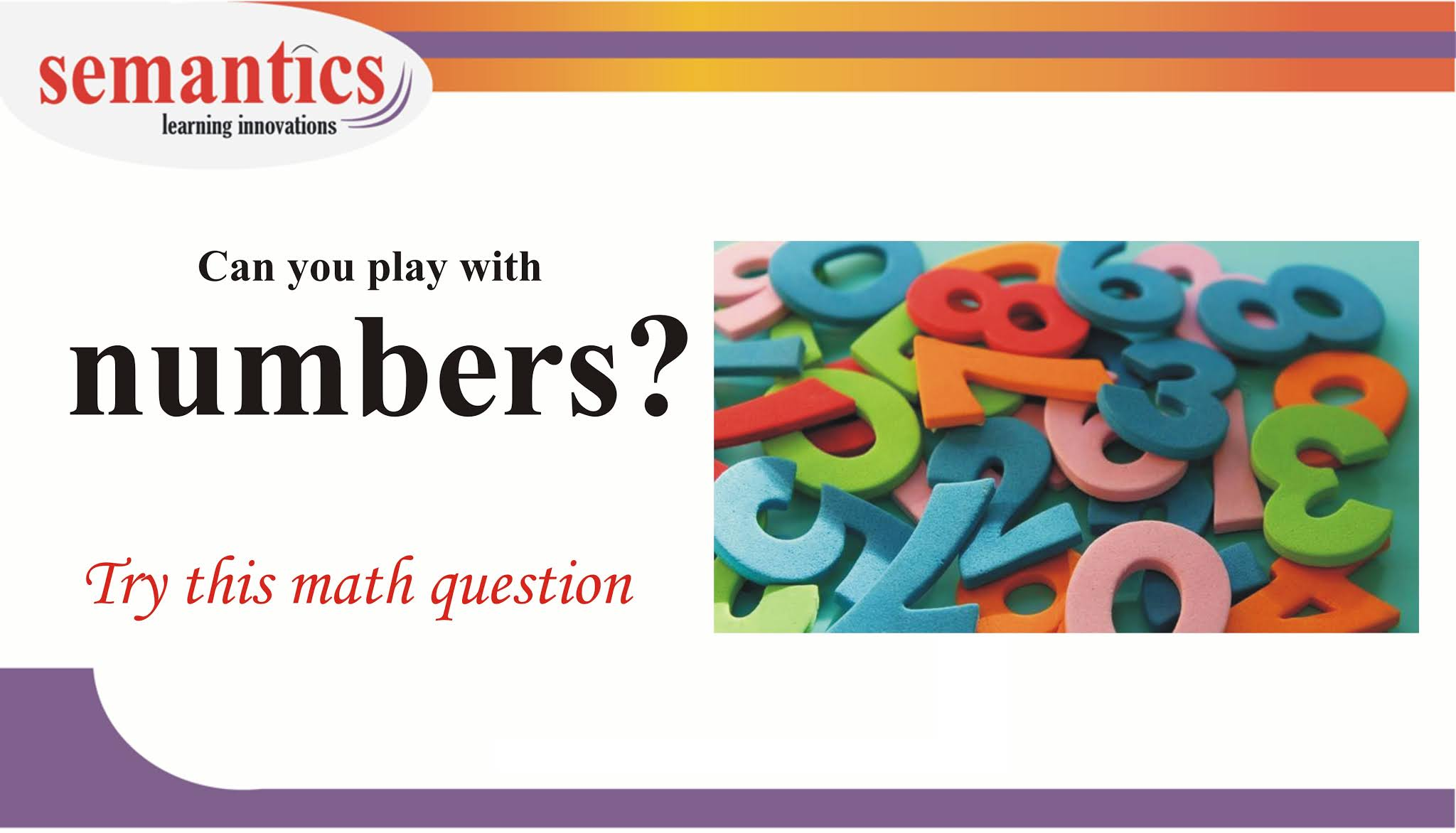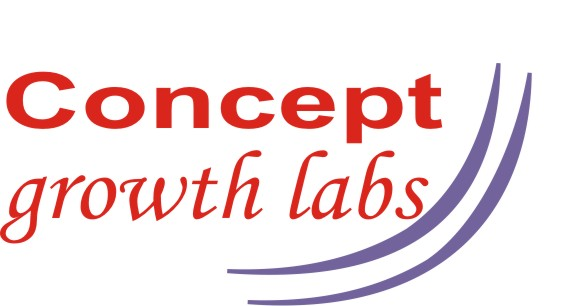# Math reasoning skills – exercise 1## Before starting this test. Click here for a demo problem Understand the thinking process.

Now take the testMath reasoning test 1

Duration 16 mins

1 / 8

If

• 0<x<4
• x can be 0 or 4.
• and y<12

Which of the following cannot be a value of  xy?

2 / 8

If x and y are prime numbers. Which of the following cannot be the sum of x and y?

3 / 8

If the quotient a/b is positive, which of the following must be true?

4 / 8

Which of the following is the product of two integers whose sum is 11?

5 / 8

The difference of two number is 2. If the sum of their reciprocals is 7/24. What is the sum of the two numbers?

6 / 8

If xy>0. Which of the following must be greater than 0?

i. x+y

ii. x2+y

iii. (x+y)2+1

iv.  (x-y)2

7 / 8

For which value of x is the following inequality true: x2 < 2x

8 / 8

The numbers a,b and c are all positive integers. a is a factor of c and b is also factor of c. if a<b , then which of the following must also be a positive integer factor of c?

0%

In-depth solutions:

Question 1 :

If xy>0. Which of the following must be greater than 0?

i.x+y          ii. x2+y          iii (x+y)2+1           iv (x-y)2

Solution:  let’s eliminate answer options by substituting values

Identify the conditions…… xy>0 and each answer option must be greater than 0. For any value of x and y the answer option >0

since xy>0.  You can substitute

1. x as +ve or y as + ve
2. x as -ve or y as – ve
3. x =y

x = -1 y = -6… xy>0 but x+y is not greater than 0. So rule out  option i and ii

x = -1 y = -6… xy>0 but x2+y  is not greater than 0. So rule out  option ii

x =1 y=1 xy>0 but (x-y)2 is not greater than 0. So rule out  option iv

—————————————————————————————————————————

Question 2:

For which value of x is the following inequality true: x2 < 2x

1.x<0       2. 0<x<2        3.-2<x<2          4. x<2         5. x>2

Solution:

Tip:  When ever you get ranges.. substitute the lowest possible value and the highest possible value. You can eliminate options faster.

Let x =-1. Hence  x2 < 2x  .. (-1)2 < 2(-1)… not true..

Hence eliminate options 1/4/3

Lets take x = 100 Hence  x2 < 2x  .. (100)2 < 2(100)… not true..

Hence eliminate options 5

—————————————————————————————————————————

Question 3:

The numbers a,b and c are all positive integers. a is a factor of c and b is also factor of c.if a<b , then which of the following must also be a positive integer factor of c?

1. a+b     2. a*b      3. c/a       4.c/(b*a)

Solution:

a is a factor of c and b is also factor of c

2 is a factor of 6 and 3 is also factor of 6

2+3 is not a factor of 6. Hence eliminate option 1

Note- take big numbers too.. don’t stick to single digit numbers

Lets take c as 100. a is 20 and b is 50

Then 100/( 20*50)  = 0.1 is not a integer factor of 100. Hence eliminate option 4

Lets take c as 24. a is 8  and b is 12

8*12 =96 is not a factor of 24. Hence eliminate option 2

—————————————————————————————————————————

Question 4:

The difference of two number is 2. If the sum of their reciprocals is 7/24. What is the sum of the two numbers?

1.20          2.14               3.15

Solution:

Let the two numbers be x and y.                 x-y=2               (1/x) + (1/y) = (7/24)

We can solve by quadratic equation. But that’s cumbersome. Lets solve by substituting numbers

(1/x) + (1/y) = (7/24)

Simplifying the fractions:  (y+x)/(xy) = 7/24

Lets break the fraction.    y+x =7              yx=24       and            x-y=2

Now lets substitute numbers .

None of the pairs satisfy all the 3 equations

Lets try again

(y+x)/(xy) = 7/24

Since this is a ratio

Lets try the next iteration of the ratio.. Multiply the numerator and denominator with 2

(y+x)/(xy) = 14/48

Lets break the fraction

y+x =14        yx=48       and      x-y=2

yx=48. Then possibilities are (24,2) (8,6),(12,4)…

8,6 satisfies all the conditions.

—————————————————————————————————————————

Question 5:

Which of the following number is the product of two integers whose sum is 11?

1. -28         2. -42          3. 12         4.26                 5. 32

Solution:

Let us assume the numbers are a and b.          a+b =11.

a and b can be positive/negative or 0.

Lets take option 1

Break 28 into prime factors….. -28 = (-1)*7*2*2

Combination of prime factors should give two numbers whose sum is 11

Possibilities are

(-14, 2) (-7,4), (14,-2)…………

None of the pairs give a difference of 11.

Lets take option 2

-42 = a*b

-42 =(-1)*2*7*3

Combination of prime factors should give two numbers whose sum is 11

(-14, 3) (-7,6), …………

-14 and 3 gives  11

Hence option 2 is the answer

—————————————————————————————————————————

Question 6:

If the quotient a/b is positive, which of the following must be true?

1. a>0            2. b>0        3. ab>0            4. a-b>0         5. a+b>0

Solution ..refer to the solution for question 3

—————————————————————————————————————————

Question 7:

If x and y are prime numbers. Which of the following cannot be the sum of x and y?

1.5     2.9      3.13     4.16       5.23

Solution ..refer to the solution for question 5

—————————————————————————————————————————

Question 8:

If 0 <= x <=4 and y<12

Which of the following cannot be a value of  xy?

1.-2         2.0            3.6          4.24         5.48

Solution .refer the solution for question 3

Hope you understood both the methods. Even if you are out of touch with math ..

With logic you can ace the math section…

If you need any help math help in your  prep… Contact me…

My contact details are here: